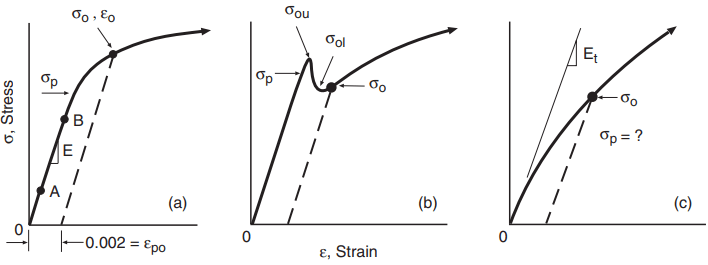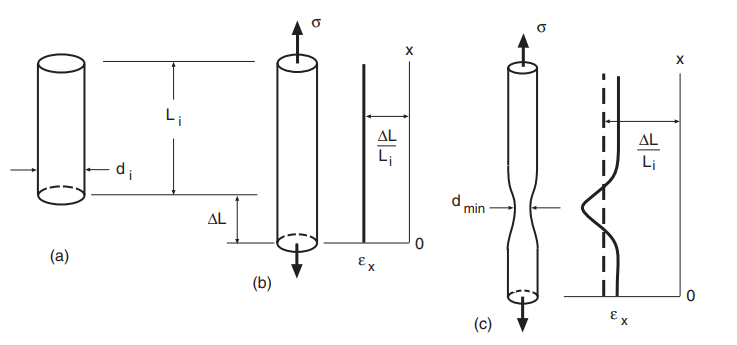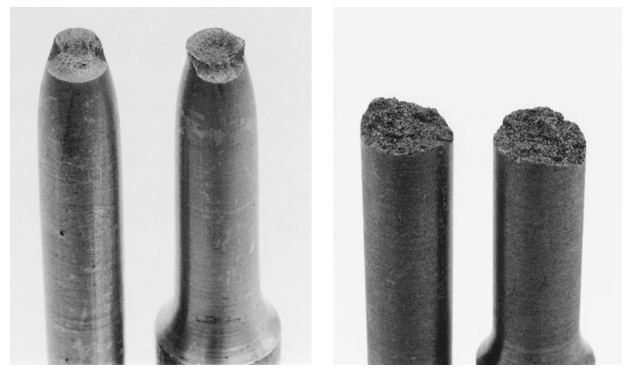# Elastic Constants

Initial portions of stress–strain curves from tension tests exhibit a variety of different behaviors for different materials as shown in Fig. 1.Figure 1 Initial portions of stress–strain curves: (a) many metals and alloys, (b) materialwith yield drop, and (c) material with no linear region.

There may be a well-defined initial straight line, as for many engineering metals, where the deformation is predominantly elastic. The elastic modulus, E, also called Young’s modulus, may then be obtained from the stresses and strains at two points on this line, such as A and B in (a):

For accuracy, the two points should be as far apart as possible, and it may be convenient to locate them on an extrapolation of the straight-line portion. Where laboratory stress–strain data are recorded at short intervals with the use of a digital computer, values judged to be on the linear portion may be fitted to a least-squares line to obtain the slope E.

If there is no well-defined linear region, a tangent modulus, Et , may be employed, which is the slope of a straight line that is tangent to the stress–strain curve at the origin, as shown in Fig. 1(c). As a practical matter, obtaining Et often involves the use of considerable judgment, so this is not a very well-defined property.

Poisson’s ratio ν can also be obtained from a tension test by measuring transverse strains during elastic behavior. Diameter measurements or a strain gage can be used for this purpose.

## Engineering Measures of Strength

The ultimate tensile strength, σu, also called simply the tensile strength, is the highest engineering stress reached prior to fracture. If the behavior is brittle, as for gray cast iron, the highest stress occurs at the point of fracture.

However, in ductile metals, the force, and hence the engineering stress, reaches a maximum and then decreases prior to fracture.

In either case, the highest force reached at any point during the test, Pmax, is used to obtain the ultimate tensile strength by dividing by the original cross-sectional area:

σu = Pmax/Ai

The engineering fracture strength, σf , is obtained from the force at fracture, Pf , even if this is not the highest force reached:

σf = Pf/Ai

Hence, for brittle materials, σu = σf , whereas for ductile materials, σu often exceeds σf .

The departure from linear-elastic behavior, as in Fig. 1, is called yielding and is of considerable interest. This is simply because stresses that cause yielding result in rapidly increasing deformation due to the contribution of plastic strain.

Any strain in excess of the elastic strain σ/E is plastic strain and is not recovered on unloading. Hence, plastic strains result in permanent deformation. Such deformation in an engineering member changes its dimensions and/or shape, which is almost always undesirable.

Thus, the first step in engineering design is usually to assure that stresses are sufficiently small that yielding does not occur, except perhaps in very small regions of a component.

The yielding event can be characterized by several methods. The simplest is to identify the stress where the first departure from linearity occurs. This is called the proportional limit, σp, and is illustrated in Fig. 1.

Some materials, as in (c), may exhibit a stress–strain curve with a gradually decreasing slope and no proportional limit. Even where there is a definite linear region, it is difficult to precisely locate where this ends. Hence, the value of the proportional limit depends on judgment, so that this is a poorly defined quantity.

Another quantity sometimes defined is the elastic limit, which is the highest stress that does not cause permanent (i.e., plastic) deformation. Determination of this quantity is difficult, as periodic unloading to check for permanent deformation is necessary.

A third approach is the offset method, which is illustrated by dashed lines in Fig. 1. A straight line is drawn parallel to the elastic slope, E or Et , but offset by an arbitrary amount. The intersection of this line with the engineering stress–strain curve is a well-defined point that is not affected by judgment, except in cases where Et is difficult to establish. This is called the offset yield strength, σo.

The most widely used and standardized offset for engineering metals is a strain of 0.002, that is, 0.2%, although other values are also used.

Note that the offset strain is a plastic strain, such as εpo = 0.002, as unloading from σo would follow a dashed line in Fig. 1, and this εpo would be the un-recovered strain.

In some engineering metals, notably in low-carbon steels, there is very little non-linearity prior to a dramatic drop in load, as illustrated in Fig. 1(b). In such cases, one can identify an upper yield point, σou, and a lower yield point, σol. The former is the highest stress reached prior to the decrease, and the latter is the lowest stress prior to a subsequent increase.

Values of the upper yield point in metals are sensitive to testing rate and to inadvertent small amounts of bending, so that reported values for a given material vary considerably. The lower yield point is generally similar to the 0.2% offset yield strength, with the latter having the advantage of being applicable to other types of stress–strain curve as well. Thus, the offset yield strength is generally the most satisfactory means of defining the yielding event for engineering metals.

For polymers, offset yield strengths are also used. However, it is more common for polymers to define a yield point only if there is an early relative maximum (upper yield point) or flat region in the curve, in which case σo is the stress where dσ/dε = 0 first occurs. In polymers with an upper yield point, σou, this stress may exceed that at fracture, σf , but in other cases, it does not.

Hence, the ultimate tensile strength σu is the higher of either σou or σf . The two situations are distinguished by describing the value as either the tensile strength at yield or the tensile strength at break.

In most materials, the proportional limit, elastic limit, and offset yield strength can be considered to be alternative measures of the beginning of permanent deformation.

However, for a nonlinear elastic material such as rubber, the first two of these measure distinctly different events, and the offset yield strength loses its significance.

## Engineering Measures of Ductility

Ductility is the ability of a material to accommodate inelastic deformation without breaking. In the case of tension loading, this means the ability to stretch by plastic strain, but with creep strain also sometimes contributing.

The engineering strain at fracture, εf , is one measure of ductility. This is usually expressed as a percentage and is then termed the percent elongation at fracture, which we will denote as 100εf . This value corresponds to the fracture point on the stress–strain curve.

The ASTM standard for tension testing of polymers includes this property, and it is given as an option in the standard for tension testing of metals. For this measurement, test standards specify the gage length as a multiple of the test section diameter or width.

For example, for specimens with round cross sections, a ratio of gage length to diameter of Li /di = 4 is specified by ASTM for use in the United States. But international standards generally employ Li /di = 5.

Another method of determining the elongation that is sometimes used for metals is to employ marks on the test section. The distance Li between these marks before testing is subtracted from the distance Lf measured after fracture. The resulting length change provides a strain value, which gives the percent elongation after fracture.

Note that the elastic strain is lost when the stress drops to zero after fracture, so this quantity is a plastic strain.

For metals with considerable ductility, the difference between 100εf and 100εpf is small, so that the distinction between these two quantities is not of great importance. However, for metals of limited ductility, the elastic strain recovered after fracture may constitute a significant fraction of εf .

Moreover, the elongation may be so small as to be difficult to measure directly from marks on the sample. It may then be useful to determine εpf from a value of εf taken from the fracture point on the stress–strain record, specifically, by subtracting the elastic strain that is estimated to be lost:

This gives an estimated after-fracture result consistent with a measurement made on a broken specimen.

Another measure of ductility is the percent reduction in area, called %RA, which is obtained by comparing the cross-sectional area after fracture, Af , with the original area:

Here, form (b) is derived from (a) as a convenience for round cross sections of initial diameter di and final diameter df . As for the elongation, a discrepancy may exist between the area after fracture and the area that existed at fracture. This presents little problem for ductile metals, but caution is needed in interpreting area reductions after fracture for polymers.

## Discussion of Necking Behavior and Ductility

If the behavior in a tension test is ductile, a phenomenon called necking usually occurs, as illustrated in Fig. 2. The deformation is uniform along the gage length early in the test, as in (a) and (b), but later begins to concentrate in one region, resulting in the diameter there decreasing more than elsewhere, as in (c).Figure 2 Deformation in a tension test of a ductile metal: (a) unstrained, (b) afteruniform elongation, and (c) during necking.

In ductile metals, necking begins at the maximum force (ultimate strength) point, and the decrease in force beyond this is a consequence of the cross-sectional area rapidly decreasing. Once necking begins, the longitudinal strain becomes non-uniform, as illustrated in (c).

Necking occurred in the steel, and to an extent in the aluminum alloy, but not in the brittle cast iron. Enlarged views show the steel and cast iron fractures in more detail in Fig. 3.Figure 3 Fractures from tension tests on 9mm diameter specimens of hot-rolled AISI 1020 steel (left) and gray cast iron (right).

The percent reduction in area is based on the minimum diameter at the fracture point and so is a measure of the highest strain along the gage length. In contrast, the percent elongation at fracture is an average over an arbitrarily chosen length.

Its value varies with the ratio of gage length to diameter, Li /di , increasing for smaller values of this ratio. As a consequence, it is necessary to standardize the gage lengths used, such as the Li /di = 4 commonly used in the United States for specimens with round cross sections, and the Li /di = 5 specified in international standards. The reduction in area is not affected by such arbitrariness and is thus a more fundamental measure of ductility than is the elongation.

## Engineering Measures of Energy Capacity

In a tension test, let the applied force be P, and let the displacement over gage length Li be
L = x. The amount of work done in deforming the specimen to a value of x = x’ is then

The volume of material in the gage length is Ai Li . Dividing both sides of the equation by this volume, and using the definitions of engineering stress and strain, gives

Hence, u is the work done per unit volume of material to reach a strain ε, and it is equal to the area under the stress–strain curve up to ε. The work done is equal to the energy absorbed by the material.

The area under the entire engineering stress–strain curve up to fracture is called the tensile toughness, uf. This is a measure of the ability of the material to absorb energy without fracture. Where there is considerable plastic strain beyond yielding, as for many engineering metals, some of the energy is stored in the microstructure of the material, but most of it is dissipated as heat.

If the stress–strain curve is relatively flat beyond yielding, then uf may be approximated as the area of a rectangle. The height is equal to the average of the yield and ultimate, and the width is equal to the fracture strain:

For materials that behave in a brittle manner, the gradually curving stress–strain response may be similar to a parabolic curve with vertex at the origin, in which case uf ≈ 2σf εf /3.

Brittle materials have low tensile toughness, despite perhaps high strength, due to low ductility. In low-strength ductile materials, the converse occurs, and the tensile toughness is also low. To have a high tensile toughness, both the strength and the ductility must be reasonably high, so that a high tensile toughness indicates a “well rounded” material.

Tensile toughness, as just defined, should not be confused with fracture toughness, which is the resistance to failure in the presence of a crack.

The tensile toughness is a useful means of comparing materials, but the fracture toughness should be considered to be the primary measure of toughness for engineering purposes.

## Strain Hardening

The rise in the stress–strain curve following yielding is described by the term strain hardening, as the material is increasing its resistance with increasing strain. A measure of the degree of strain hardening is the ratio of the ultimate tensile strength to the yield strength.

Hence, we define the strain hardening ratio = σuo. Values of this ratio above about 1.4 are considered relatively high for metals, and those below 1.2 relatively low.

## Related Articles

Categories: Material Science